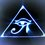# Cubics

A cubic polynomial is a polynomial in the form of $$y=(ac)x^3+(ad+bc)x^2+(ae+bd)x+(be)$$, where ac cannot equal zero. It can be factored into the form $(ax+b)(cx^2+dx+e)$.

Some of their noteworthy properties include:

• It has a degree of three.
• Its common difference is the third difference.
• The end behavior of the graph is that of an odd function.

End Behavior Of Cubics

The value of ac in the equation is the determining factor in its end behavior.

If ac is positive we can say:

• As x approaches positive infinity y approaches positive infinity .
• As x approaches negative infinity y approaches negative infinity.

If ac is negative we can say:

• As x approaches positive infinity y approaches negative infinity.
• As x approaches negative infinity y approaches positive infinity.

* Factoring Cubics*

Cubics can be factored as the product of a quadratic and a binomial. The quadratic can be factored further. To see How to do that you can check out quadratic factoring. You can do this with inspection or by long division.

Inspection

Factor the equation $y= 2x^3 +10x^2+18x+18$

Knowing that the equation $y=(ac)x^3+(ad+bc)x^2+(ae+bd)x+be$ can be factored as $(ax+b)(cx+dx+e)$ we can define the coefficients of the terms as well as the constant term.

$2x^3 =(ac)x^3$

$10x^2=(ad+bc)x^2$

$18x=(ae+bd)x$

$18=eb$

Now we can use this knowledge to test possible values for the variables and decide which ones are the factors. In this particular case the factored form of the equation is :

$(1x+3)(2x^2+4x+6)$

Long Division

Factor the equation $y= x^3 +8x^2+22x+35)$

We know that the equation $y=(ac)x^3+(ad+bc)x^2+(ae+bd)x+be$ can be factored as the product of a binomial and a trinomial. If this is the case we can divide it by the binomial and resulting quotient is the trinomial factor. So we can infer that the constant term of the equation is a multiple of the constant term in the binomial.

If the factors are $(x+b)(x^2+dx+e)$, * eb* =35

The factors of 35 are 35, 7, 5, and 1. These are the possible values for e and *b

Now If we divide $y= x^3 +8x^2+22x+35)$ by $(x+b)$ substituting the possible values for b we will find another factor.

As a result we find the factors of the equation to be:

$(x+5)(x^2+3x+7)$Note by Brody Acquilano
5 years, 7 months ago

This discussion board is a place to discuss our Daily Challenges and the math and science related to those challenges. Explanations are more than just a solution — they should explain the steps and thinking strategies that you used to obtain the solution. Comments should further the discussion of math and science.

When posting on Brilliant:

• Use the emojis to react to an explanation, whether you're congratulating a job well done , or just really confused .
• Ask specific questions about the challenge or the steps in somebody's explanation. Well-posed questions can add a lot to the discussion, but posting "I don't understand!" doesn't help anyone.
• Try to contribute something new to the discussion, whether it is an extension, generalization or other idea related to the challenge.

MarkdownAppears as
*italics* or _italics_ italics
**bold** or __bold__ bold
- bulleted- list
• bulleted
• list
1. numbered2. list
1. numbered
2. list
Note: you must add a full line of space before and after lists for them to show up correctly
paragraph 1paragraph 2

paragraph 1

paragraph 2

[example link](https://brilliant.org)example link
> This is a quote
This is a quote
    # I indented these lines
# 4 spaces, and now they show
# up as a code block.

print "hello world"
# I indented these lines
# 4 spaces, and now they show
# up as a code block.

print "hello world"
MathAppears as
Remember to wrap math in $$ ... $$ or $ ... $ to ensure proper formatting.
2 \times 3 $2 \times 3$
2^{34} $2^{34}$
a_{i-1} $a_{i-1}$
\frac{2}{3} $\frac{2}{3}$
\sqrt{2} $\sqrt{2}$
\sum_{i=1}^3 $\sum_{i=1}^3$
\sin \theta $\sin \theta$
\boxed{123} $\boxed{123}$

Sort by:

ahh yes the factors of 35 would be 35,7,5,1 (positive or negative)

- 5 years, 7 months ago

You have to account for negative factors as well.

- 5 years, 7 months ago